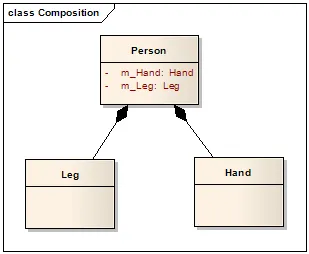# Programming - Java Composition and ArrayList# Composition:

Last time without even noticing we did some kind of Java Composition. We had our Driverclass create and use an instance of an object of type Student that was an Objectclass. This exactly is what a Composition is. We implement the has-a relationship by having a class contain other classes in form of objects that can be simple variables, Arrays and so on. For example a Human has 2 Legs and 2 Hands so we could have a class called "Human" that contains 4 other classes inside in form of Objects: 2 "Leg" classes and 2 "Hand" classes for the left and right one individually. So, we can see that not only the Driverclass uses Objects of Classes, but sometimes even Objectclasses contain other Classes to make the Code reusable and easier understandable.

# ArrayList:

An ArrayList is an Object that is inside of the java.util library and can be used for making dynamic Arrays or Lists. Like we know from the most languages, an Array is not dynamic, but static and you can only make it dynamic when allocating memory running the Code, and unlike C, Java has no memory allocation functions for Arrays and that's why we use a List. We could use a Stack or Heap, that we will talk about in C this week, but these types of Data structures have also an limitation in space and that's why we use a List. There is a Object called List also, but we will use the ArrayList, because it contains many predefined useful functions that make it easier to Code.

To use an ArrayList you need to import the java.util.ArrayList class and then you have to write this line of Code to create one:

``ArrayList<variable_type> list_name = new ArrayList<variable_type>();``

Then using:

• list_name.get(i) you can get the item in index "i"

You can just press . after list_name to see all of the predefined functions that could sort the Array, check if it is empty, return the size and much more.

# Code:

Let's create a Code that contains 3 Classes: 1 Driverclass called Driver and 2 Objects called Student and Course from which a Course-ArrayList will be a part of each Student. A Student has an Integer id, a String name, a String surname and the ArrayList of Coures. A Course has an Integer id, the String name and an double grade. The Driverclass will create an ArrayList of Students and we will add one Student and 2 Courses to his ArrayList. Afterwards, we will print those in the Console. We will create Constructors and the toString() method, but the variables will be "default" and not private and so no Getters-Setters are needed to make it easier to write and have smaller lines of Code.

Course:

``public class Course { // Course.java``
`` int id;``
`` String name;``
`` double grade;``

`` public Course(int id, String name, double grade) { //overloaded constructor``
``     this.id = id;``
``     this.name = name;``
``     this.grade = grade;``
`` }``

`` @Override``
`` public String toString() {``
``     return id + " " + name + " " + grade;``
`` }``
``}``

Student:

``import java.util.ArrayList; // ArrayList Library``
``public class Student { // Student.java``
`` int id;``
`` String name;``
`` String surname;``
`` // a Student HAS a Course ArrayList in it``
`` ArrayList<Course> courses = new ArrayList<Course>(); // ArrayList of Course``

`` //pseudo-overloaded constructor (no ArrayList``
`` public Student(int id, String name, String surname) { ``
``     this.id = id;``
``     this.name = name;``
``     this.surname = surname;``
`` }``

`` @Override``
`` public String toString() {``
``     String temp = "";// temporary string``
``     temp += id + " ";``
``     temp += name + " ";``
``     temp += surname + "\n";``
``     ``
``     // we will add all of the courses inside of the string``
``     for (int i = 0; i < courses.size() - 1; i++) { // size() is the length ``
``                                                     // of ArrayList courses``
``         temp += courses.get(i) + "\n";``

``     }``
``     temp += courses.get(courses.size() - 1);``
``     return temp; // we return a String representation of the Student``

`` }``
``}``

TestStudent: (instead of Driver we use also TestObject_name sometimes)

``import java.util.ArrayList;``
``public class TestStudent { // TestStudent.java``

`` public static void main(String[] args) {``
``     ArrayList<Student> students = new ArrayList<>(); // Dynamic ArrayList of Student Objects``

``     students.add(new Student(569, "Nick", "Peterson")); // with add you put a new item in the array``
``     students.get(0).courses.add(new Course(669, "Physics", 7.5)); // with get you get the index of the array``
``     students.get(0).courses.add(new Course(665, "Chemistry", 9));``

``     //print every Student in the Array (we have only one here)``
``     for (int i = 0; i < students.size(); i++) { //size() is the length of ArrayList students``
``         System.out.println(students.get(i));``
``     }``

`` }``

``}``

H2
H3
H4
3 columns
2 columns
1 column